Area of segment of circle and length of arc

Chapter 11 Class 10 Areas related to Circles
Concept wise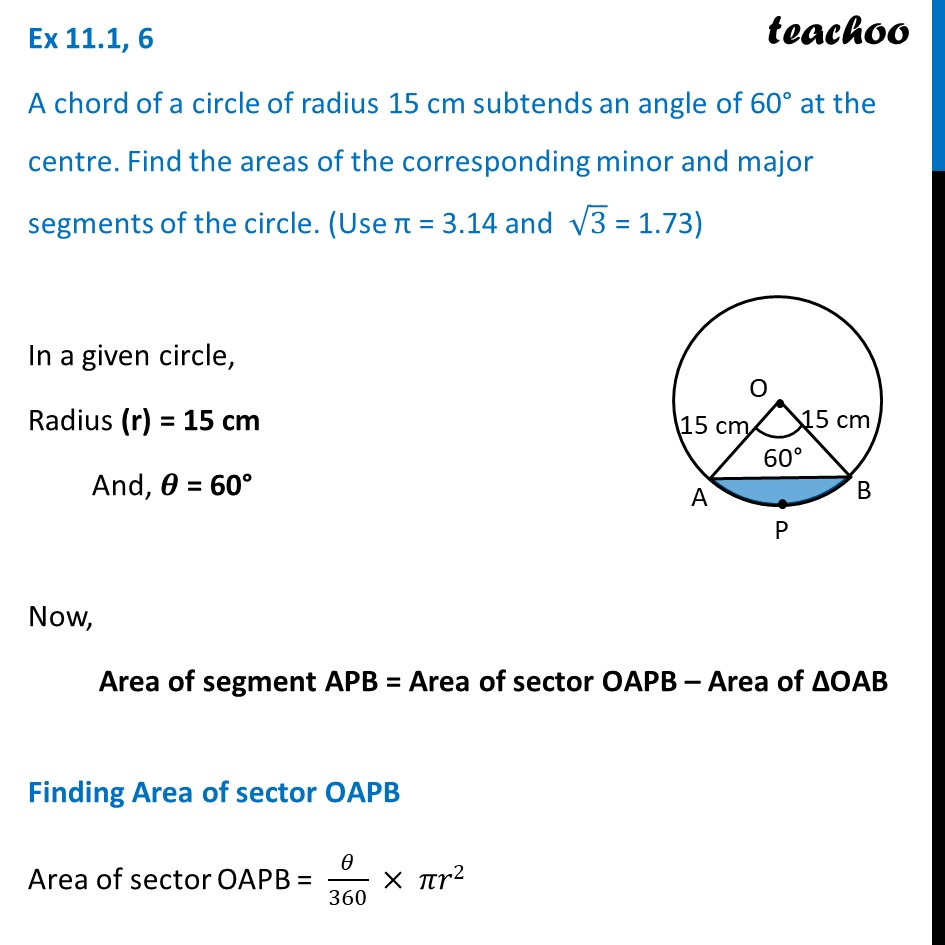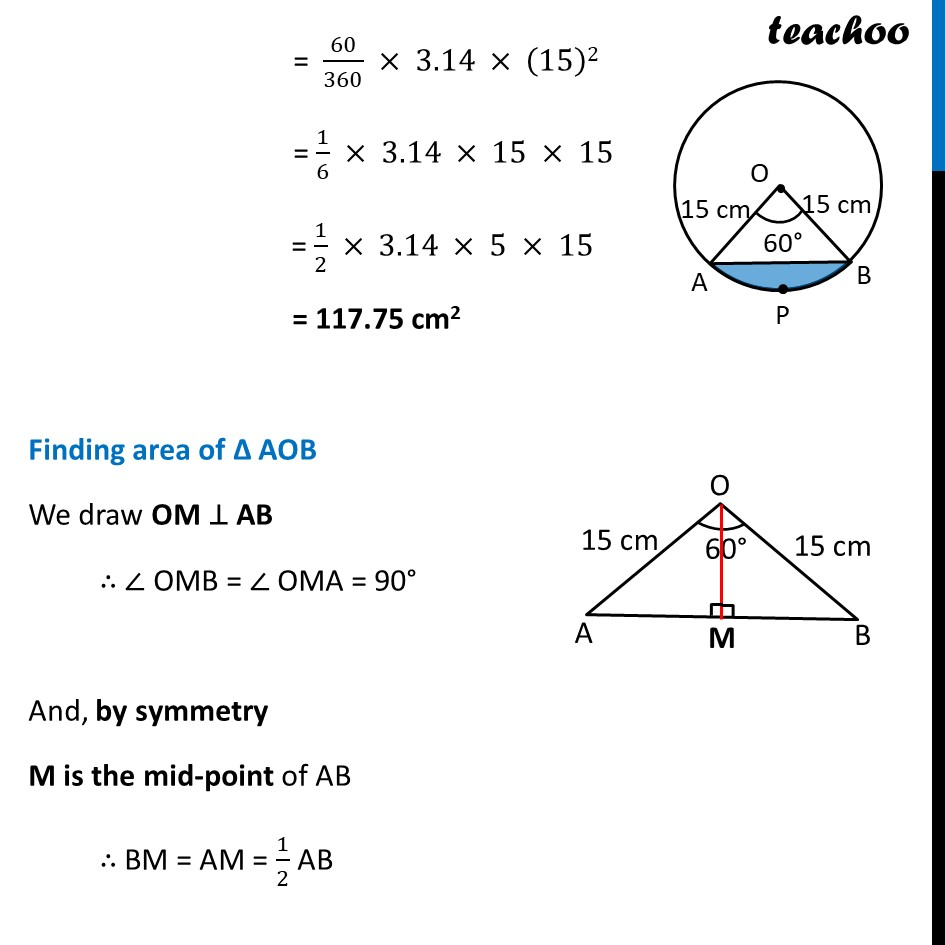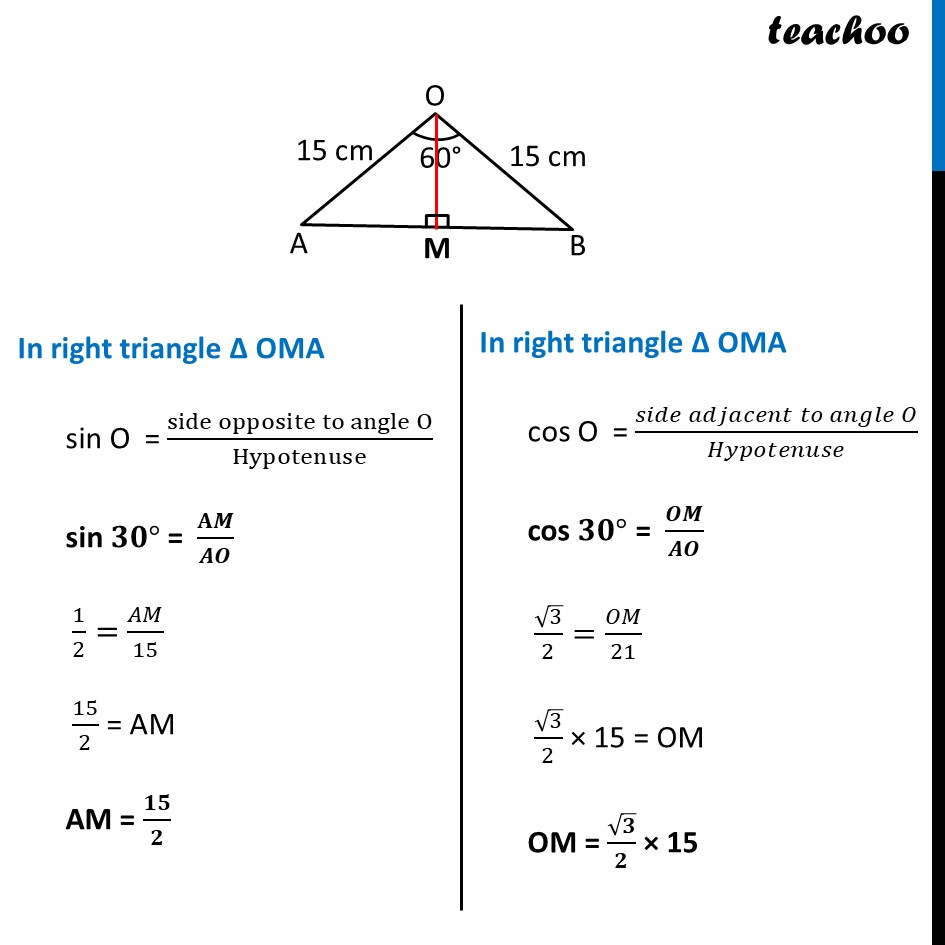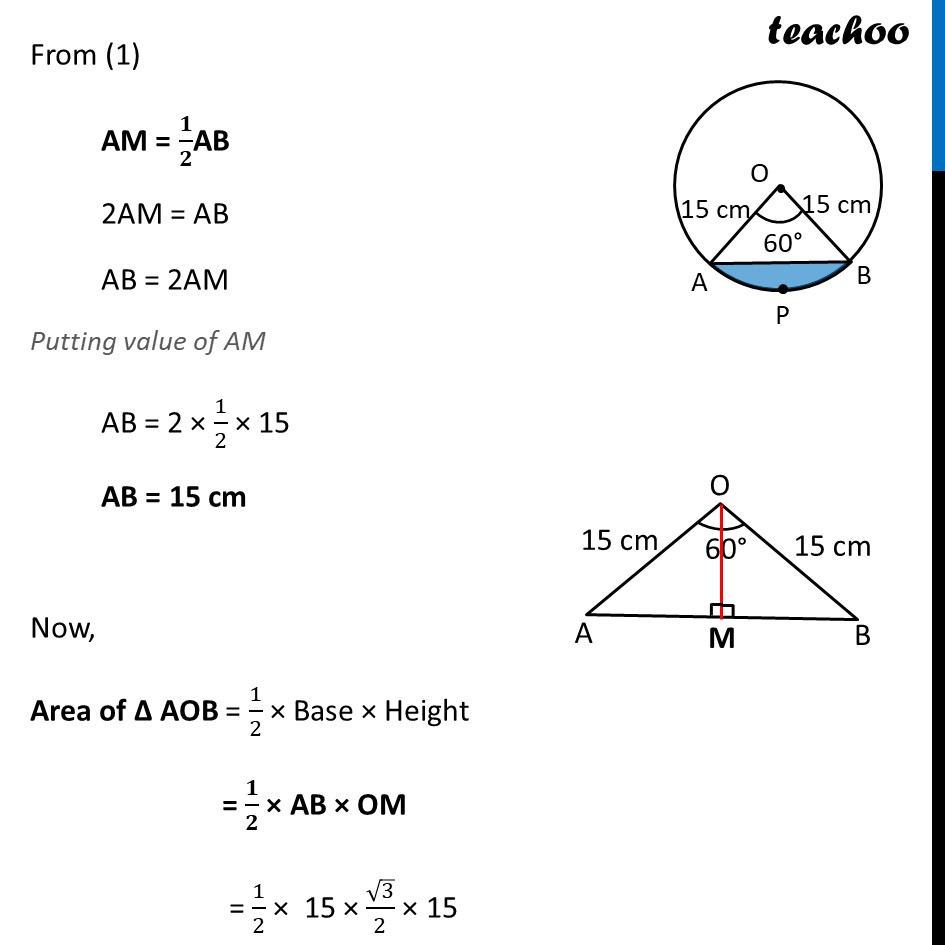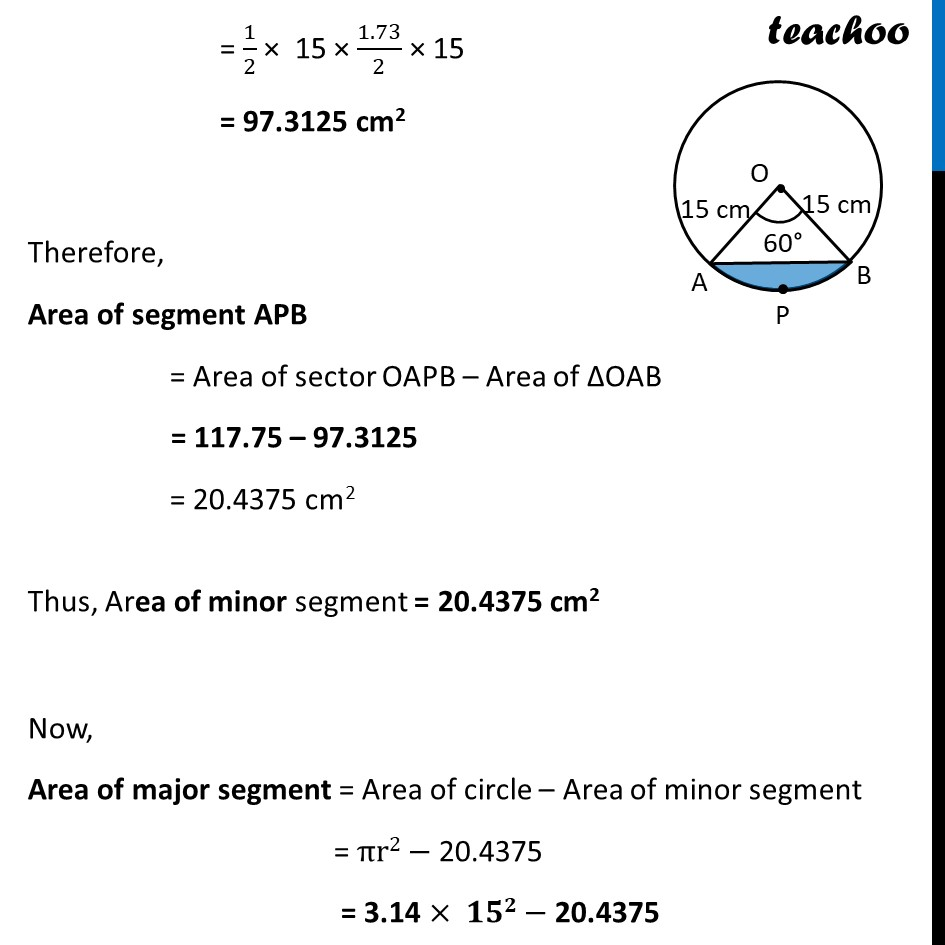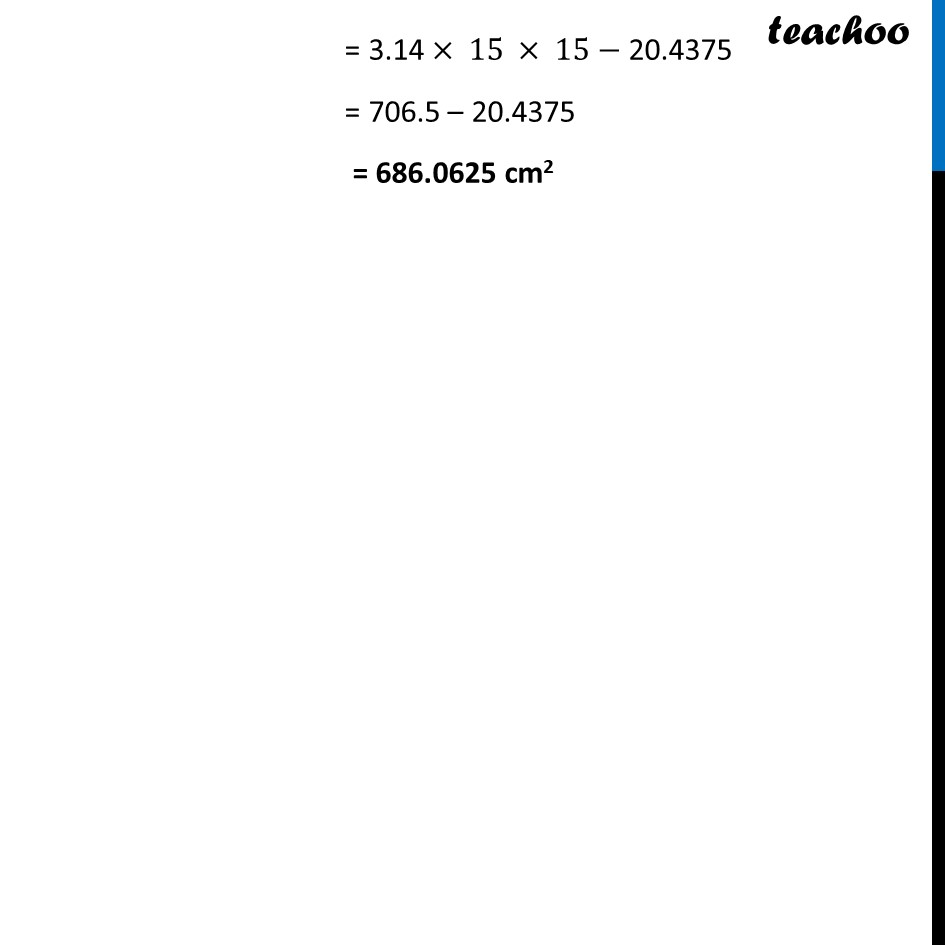Learn in your speed, with individual attention - Teachoo Maths 1-on-1 Class

### Transcript

Ex 11.1, 6 A chord of a circle of radius 15 cm subtends an angle of 60° at the centre. Find the areas of the corresponding minor and major segments of the circle. (Use π = 3.14 and √3 = 1.73) In a given circle, Radius (r) = 15 cm And, 𝜽 = 60° Now, Area of segment APB = Area of sector OAPB – Area of ΔOAB Finding Area of sector OAPB Area of sector OAPB = 𝜃/360 × 𝜋𝑟2 = 60/360 × 3.14 × (15)2 = 1/6 × 3.14 × 15 × 15 = 1/2 × 3.14 × 5 × 15 = 117.75 cm2 Finding area of Δ AOB We draw OM ⊥ AB ∴ ∠ OMB = ∠ OMA = 90° And, by symmetry M is the mid-point of AB ∴ BM = AM = 1/2 AB In right triangle Δ OMA sin O = (side opposite to angle O)/Hypotenuse sin 𝟑𝟎° = 𝐀𝑴/𝑨𝑶 1/2=𝐴𝑀/15 15/2 = AM AM = 𝟏𝟓/𝟐 In right triangle Δ OMA cos O = (𝑠𝑖𝑑𝑒 𝑎𝑑𝑗𝑎𝑐𝑒𝑛𝑡 𝑡𝑜 𝑎𝑛𝑔𝑙𝑒 𝑂)/𝐻𝑦𝑝𝑜𝑡𝑒𝑛𝑢𝑠𝑒 cos 𝟑𝟎° = 𝑶𝑴/𝑨𝑶 √3/2=𝑂𝑀/21 √3/2 × 15 = OM OM = √𝟑/𝟐 × 15 From (1) AM = 𝟏/𝟐AB 2AM = AB AB = 2AM Putting value of AM AB = 2 × 1/2 × 15 AB = 15 cm Now, Area of Δ AOB = 1/2 × Base × Height = 𝟏/𝟐 × AB × OM = 1/2 × 15 × √3/2 × 15 = 1/2 × 15 × 1.73/2 × 15 = 97.3125 cm2 Therefore, Area of segment APB = Area of sector OAPB – Area of ΔOAB = 117.75 – 97.3125 = 20.4375 cm2 Thus, Area of minor segment = 20.4375 cm2 Now, Area of major segment = Area of circle – Area of minor segment = πr2− 20.4375 = 3.14 × 𝟏𝟓𝟐− 20.4375 = 3.14 × 15 × 15−"20.4375" = 706.5 – 20.4375 = 686.0625 cm2